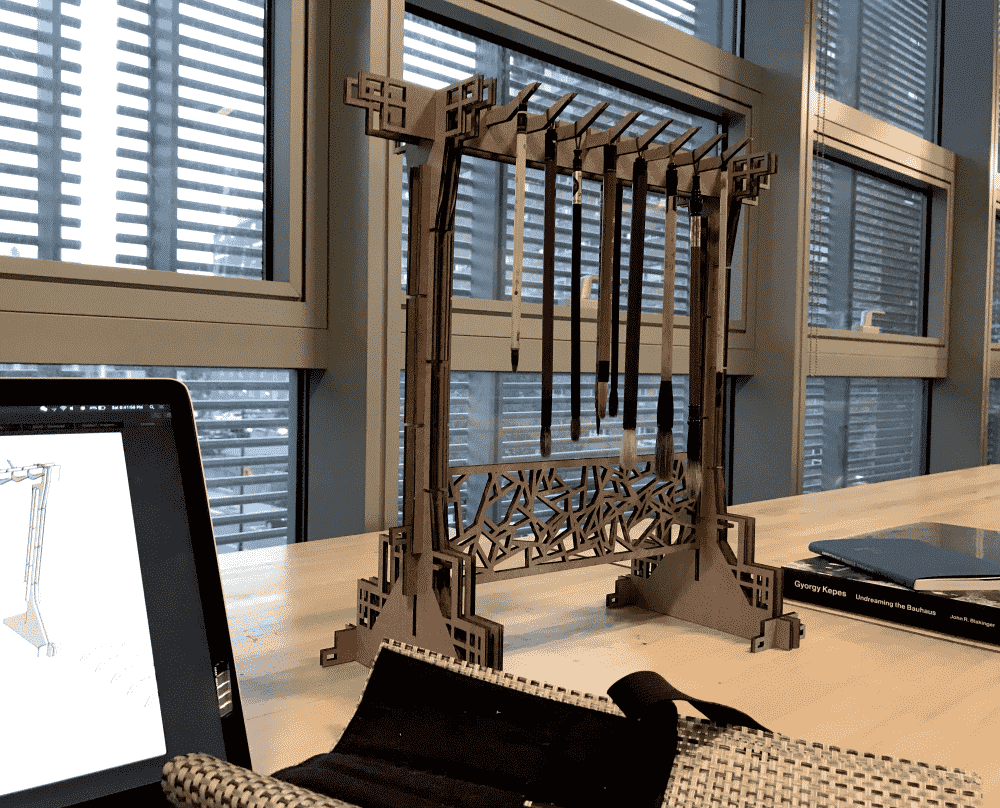# Laser Cutter Construction Kit

For the “construction kit” assignment, I decided to make a brush stand to hang my calligraphy brushes. Chinese calligraphy brushes have delicate bristles easily deformed, so it's best to let them dangle in mid-air.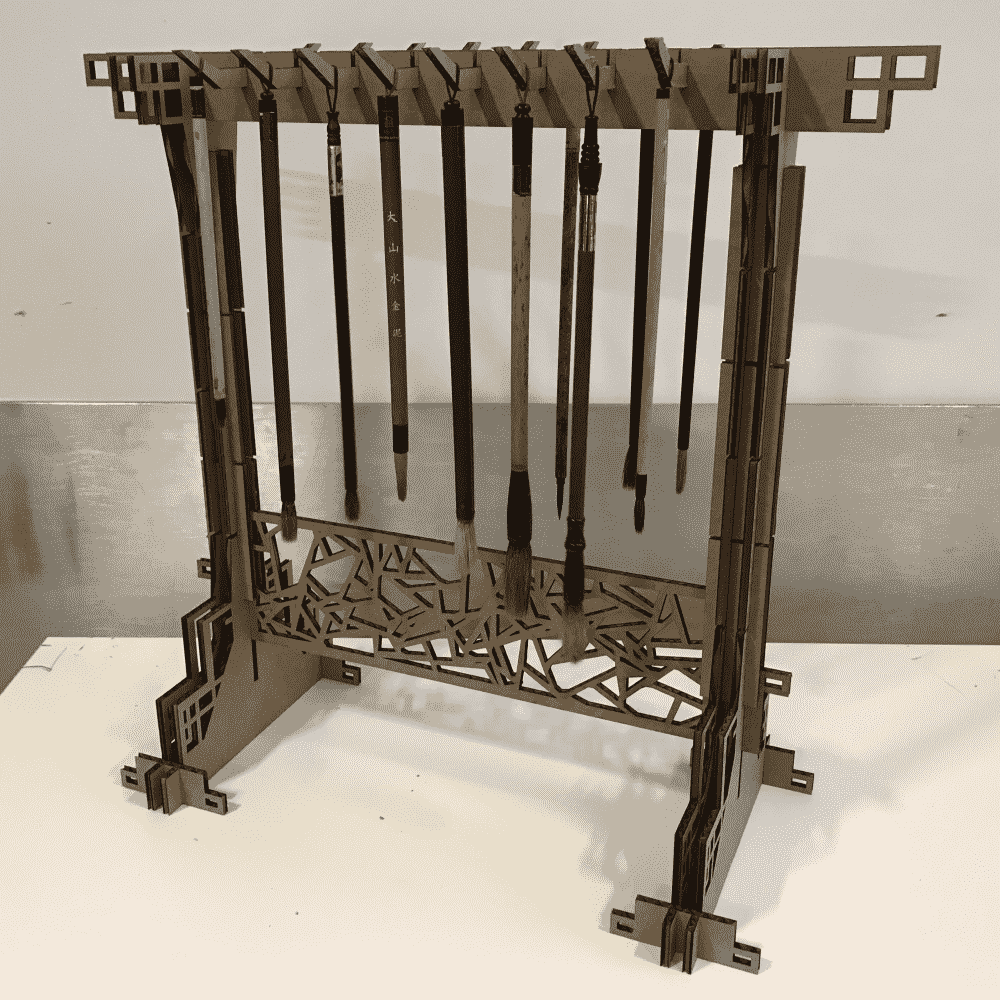Initially I decided to learn FreeCAD. Discovering the GUI to be terrible, I decided to learn FreeCAD's python API instead. However, that turned out terrible too. As I ran out of time, I decided to stick to what I know -- write a JS program from scratch to generate the entire design.

The great thing about writing program from scratch is that it's incredibly parametric. Everything is controlled by variables. (You can check out an online demo of the final program here).

I first generated a “plain” version without any decorations: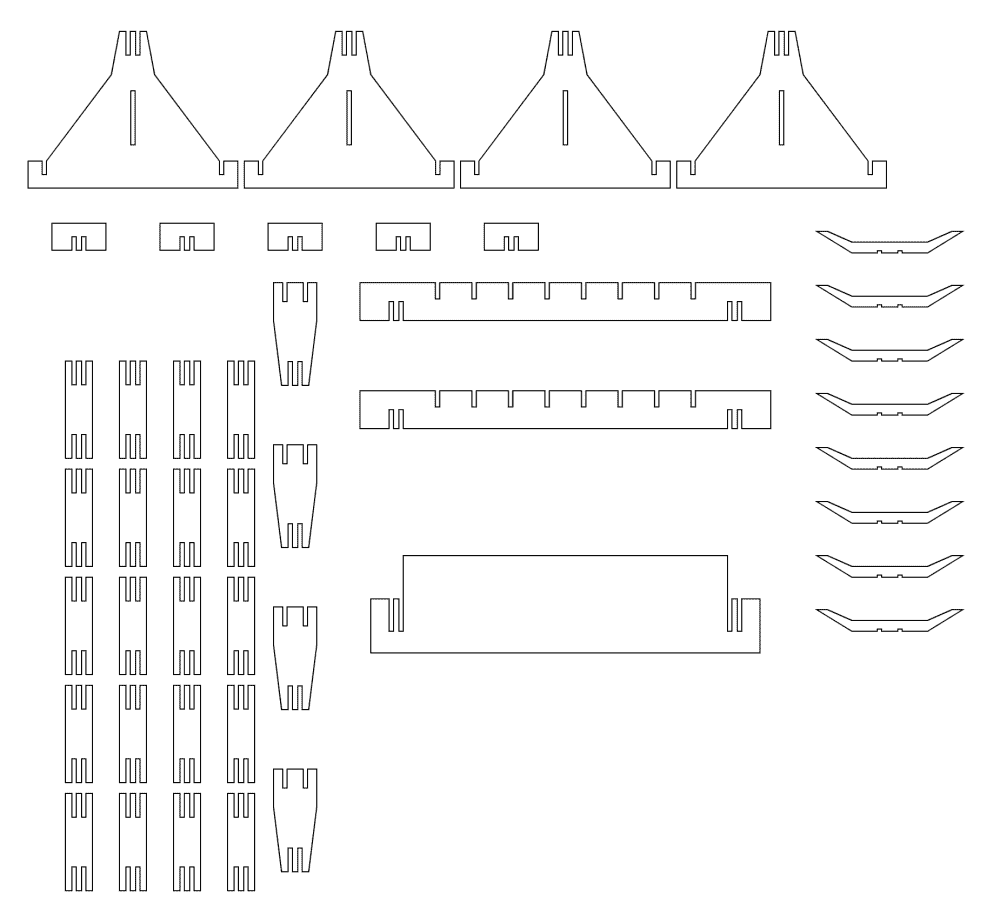While designing the structure, I feared that cardboard being a sloppy material would make my brush stand too janky and unable to stand the weight of brushes. So everywhere I considered how I could make the structure more robust by reasoning about the forces.

So far I've been only imagining how it would look like when assembled in my mind. I thought it'd be a good idea to try it out in a 3D modelling software. So I wrote a ruby script for SketchUp to import my JS output, automatically extrude the shapes and build components. Then I manually assembled the pieces in SketchUp:

require 'sketchup.rb'
require 'extensions.rb'
require 'langhandler.rb'
thick = 4.1
data = [] # PASTE POLYLINES DATA HERE!

model = Sketchup.active_model
entities = model.active_entities

data.each_with_index do |pts,i|
puts i
begin
f.pushpull(thick);
rescue
puts ":P"
end
end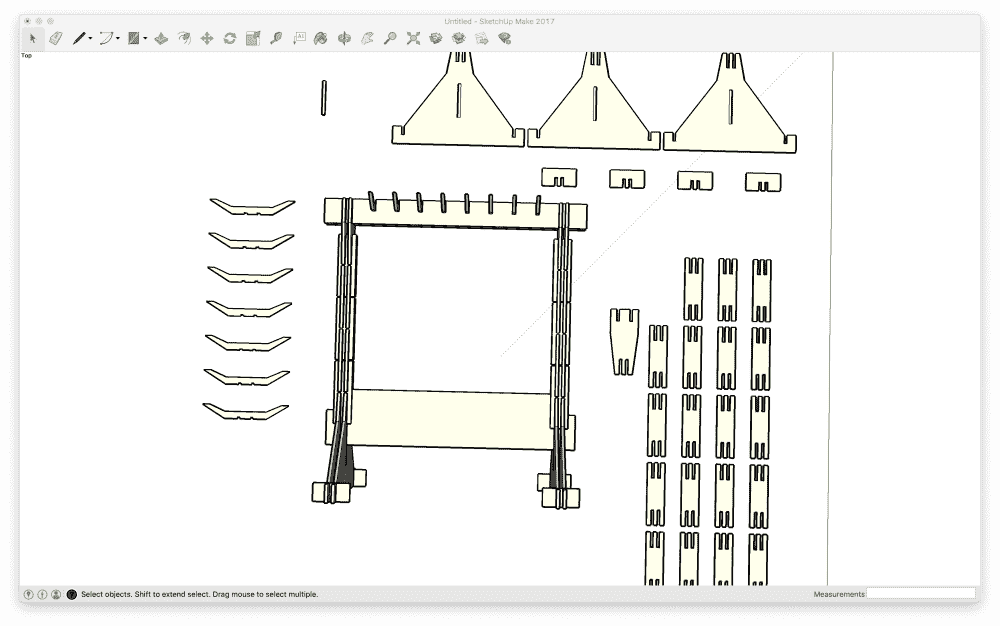Seemed OK. So I started adding decorations. I thought it was important to add some intricate patterns. This way I can exploit the precision of the laser cutter; otherwise I might as well scissor the simple shapes by hand.

At first I was more ambitious about the patterns, but my signed up laser cutter time slot is drawing near, and I had to whip up something quick. I decided to recreate those classical “shattered ceramic” aesthetics. This way I could generate randomly instead of painstakingly entering coordinates in my program. The obvious algorithm was to use voronoi. However, I observed that the classical patterns have sharper corners, whereas voronoi tends to create rounded cells. I came up with a custom algorithm involving line intersection and recursive growing. The result looked kinda OK. If given more time I could probably improve it.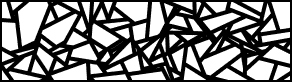For the pattern near the “legs” of the stand, I used an even lazier generative procedure: just a bunch of rectangle frames stacked on top of each other. For other decorations of smaller sizes, I coded the shapes manually without randomness. Now the design looked much more interesting: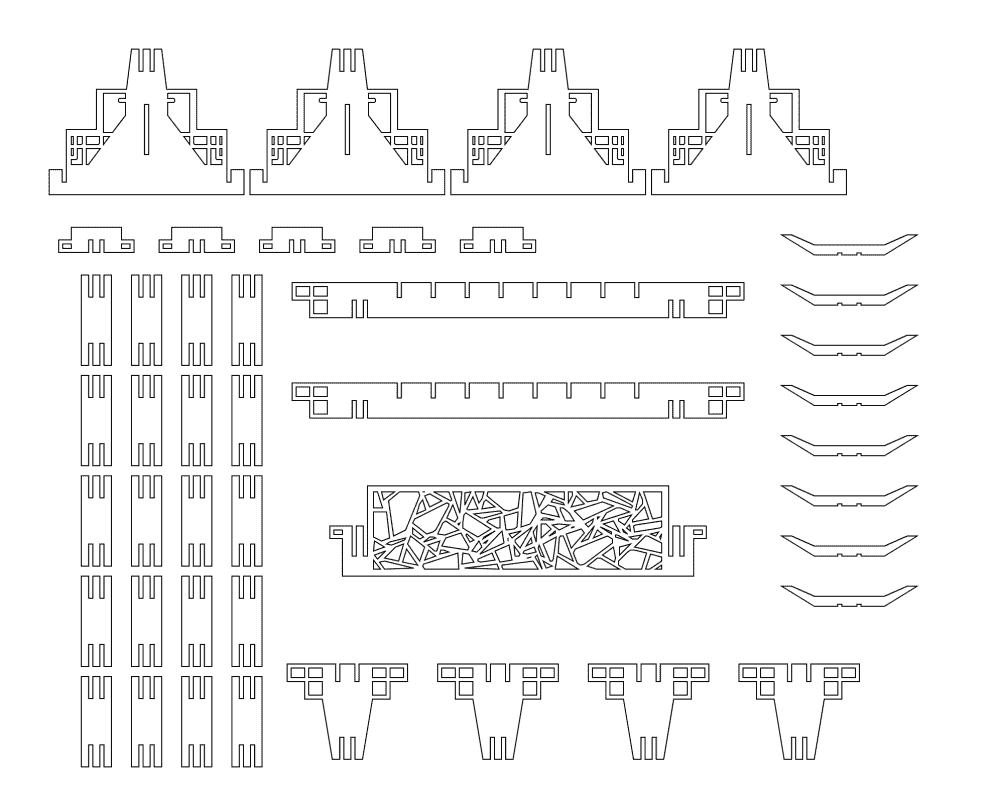Below is the source code for generating the final design (~600 lines; please scroll; run online here):

var topw = 300;
var toph = 35;
var topd = 50;
var topp = 40;
var topdsp = 15;
var topjh = 60;
var slotw = 4.1;
var ntopslot = 8;
var topsloth = 15;
var colw = 30;
var colh = 90;
var colsloth = 22;
var colsp = 7;
var botd0 = 40;
var botd1 = 160;
var botd2 = 194;
var botjw = 50;
var both0 = 40;
var both1 = 80;
var both2 = 25;
var botstx = 0.5;
var botsty = 0.5;
var hookd0 = 70;
var hookd1 = 115;
var hookd2 = 135;
var hookh = 10;
var hooksloth = 2;
var hookdh = 10;
var banjh = 50;
var banjsloth= 30;
var botbanjsh = 15;
var banh = 90;
var banp = 25;
var patw = 4;

let findContours, approxPolyDP;

if (typeof require != 'undefined'){
({findContours, approxPolyDP} = require('./findcontours.js'));
}else{
({findContours, approxPolyDP} = FindContours);
window.createCanvas = function(w,h){
let cnv = document.createElement('canvas');
cnv.width = w;
cnv.height = h;
return cnv;
}
}

let jsr = 0x5EED;
function rand(){
jsr^=(jsr<<17);
jsr^=(jsr>>13);
jsr^=(jsr<<5);
return (jsr>>>0)/4294967295;
}

function seg_isect(p0x, p0y, p1x, p1y, q0x, q0y, q1x, q1y, is_ray = false) {
let d0x = p1x - p0x;
let d0y = p1y - p0y;
let d1x = q1x - q0x;
let d1y = q1y - q0y;
let vc = d0x * d1y - d0y * d1x;
if (vc == 0) {
return null;
}
let vcn = vc * vc;
let q0x_p0x = q0x - p0x;
let q0y_p0y = q0y - p0y;
let vc_vcn = vc / vcn;
let t = (q0x_p0x * d1y - q0y_p0y * d1x) * vc_vcn;
let s = (q0x_p0x * d0y - q0y_p0y * d0x) * vc_vcn;
if (0 <= t && (is_ray || t < 1) && 0 <= s && s < 1) {
return t;
}
return null;
}

function toppart(){
let ps = [];
ps.push([-topw/2-topp-toph,-toph/2]);
let sp = (topw - ntopslot * slotw)/(ntopslot+1);
let x = -topw/2+sp;
for (let i = 0; i < ntopslot; i ++){
ps.push([x,-toph/2],[x,-toph/2+topsloth],[x+slotw,-toph/2+topsloth],[x+slotw,-toph/2]);
x += slotw + sp;
}
ps.push([topw/2+topp+toph,-toph/2]);
ps.push([topw/2+topp+toph,0]);
ps.push([topw/2+topp+toph/2,0]);
ps.push([topw/2+topp+toph/2,toph/2]);
// ps.push([topw/2+topp,toph/2]);

let a = slotw + colsp;
ps.push([topw/2+a+slotw,toph/2]);
ps.push([topw/2+a+slotw,0]);
ps.push([topw/2+a,0]);
ps.push([topw/2+a,toph/2]);

ps.push([topw/2+slotw,toph/2]);
ps.push([topw/2+slotw,0]);
ps.push([topw/2,0]);
ps.push([topw/2,toph/2]);

ps.push([-topw/2,toph/2]);
ps.push([-topw/2,0]);
ps.push([-topw/2-slotw,0]);
ps.push([-topw/2-slotw,toph/2]);

ps.push([-topw/2-a,toph/2]);
ps.push([-topw/2-a,0]);
ps.push([-topw/2-a-slotw,0]);
ps.push([-topw/2-a-slotw,toph/2]);

// ps.push([-topw/2-topp,toph/2]);
ps.push([-topw/2-topp-toph/2,toph/2]);

ps.push([-topw/2-topp-toph/2,0]);
ps.push([-topw/2-topp-toph,0]);

let pps = [ps];

return pps;
}

function topjoint(){
let ps = [];
let l = (topd-topdsp-slotw-slotw)/2;

ps.push([-topd/2-toph,-toph/2]);
ps.push([-topd/2+l,-toph/2]);
ps.push([-topd/2+l,-toph/2+toph/2]);
ps.push([-topd/2+l+slotw,-toph/2+toph/2]);
ps.push([-topd/2+l+slotw,-toph/2]);

l += slotw + topdsp;

ps.push([-topd/2+l,-toph/2]);
ps.push([-topd/2+l,-toph/2+toph/2]);
ps.push([-topd/2+l+slotw,-toph/2+toph/2]);
ps.push([-topd/2+l+slotw,-toph/2]);

// ps.push([topd/2,-toph/2]);
//

ps.push([topd/2+toph,-toph/2]);
ps.push([topd/2+toph,0]);
ps.push([topd/2+toph/2,0]);
ps.push([topd/2+toph/2,toph/2]);

ps.push([topd/2,toph/2]);

ps.push([colw/2,toph/2+topjh]);

let s = (colw-colsp-slotw-slotw)/2;

ps.push([colw/2-s,toph/2+topjh]);
ps.push([colw/2-s,toph/2+topjh-colsloth]);
ps.push([colw/2-s-slotw,toph/2+topjh-colsloth]);
ps.push([colw/2-s-slotw,toph/2+topjh]);

s += slotw + colsp;
ps.push([colw/2-s,toph/2+topjh]);
ps.push([colw/2-s,toph/2+topjh-colsloth]);
ps.push([colw/2-s-slotw,toph/2+topjh-colsloth]);
ps.push([colw/2-s-slotw,toph/2+topjh]);

ps.push([-colw/2,toph/2+topjh]);
ps.push([-topd/2,toph/2]);

ps.push([-topd/2-toph/2,toph/2])
ps.push([-topd/2-toph/2,0]);
ps.push([-topd/2-toph,0]);

let pps = [ps];

return pps;
}

function coljoint(){
let ps = [];
let s = (colw-colsp-slotw-slotw)/2;

ps.push([-colw/2,-colh/2]);

ps.push([-colw/2+s,-colh/2]);
ps.push([-colw/2+s,-colh/2+colsloth]);
ps.push([-colw/2+s+slotw,-colh/2+colsloth]);
ps.push([-colw/2+s+slotw,-colh/2]);

s += slotw + colsp;
ps.push([-colw/2+s,-colh/2]);
ps.push([-colw/2+s,-colh/2+colsloth]);
ps.push([-colw/2+s+slotw,-colh/2+colsloth]);
ps.push([-colw/2+s+slotw,-colh/2]);

ps.push([colw/2,-colh/2]);
ps.push([colw/2,colh/2]);

s = (colw-colsp-slotw-slotw)/2;

ps.push([colw/2-s,colh/2]);
ps.push([colw/2-s,colh/2-colsloth]);
ps.push([colw/2-s-slotw,colh/2-colsloth]);
ps.push([colw/2-s-slotw,colh/2]);

s += slotw + colsp;
ps.push([colw/2-s,colh/2]);
ps.push([colw/2-s,colh/2-colsloth]);
ps.push([colw/2-s-slotw,colh/2-colsloth]);
ps.push([colw/2-s-slotw,colh/2]);

ps.push([-colw/2,colh/2]);
ps.push([-colw/2,-colh/2]);
return [ps];
}

function botpattern(){
const cnv = createCanvas((botd1-botd0)/2,both1);
const ctx = cnv.getContext('2d');
ctx.fillStyle="white";
ctx.strokeStyle = "black";
ctx.fillRect(0,0,cnv.width,cnv.height);
ctx.lineWidth = patw*2;

ctx.beginPath();
ctx.moveTo(0,0);
ctx.lineTo(cnv.width*botstx,0);
ctx.lineTo(cnv.width*botstx,cnv.height*botsty);
ctx.lineTo(cnv.width,cnv.height*botsty);
ctx.lineTo(cnv.width,cnv.height);
ctx.lineTo(0,cnv.height);
ctx.stroke();
ctx.lineWidth = patw;

// ctx.strokeRect(0,0,cnv.width*botstx,cnv.height);
// ctx.strokeRect(0,cnv.height*botsty,cnv.width,cnv.height*(1-botsty));

for (let i = 0; i < 8; i++){
// ctx.strokeRect(0,cnv.height,~~(rand()*7)*cnv.width/7,-(~~(rand()*7)*cnv.height/7));
// ctx.strokeRect(rand()*cnv.width,rand()*cnv.height,rand()*cnv.width,rand()*cnv.height);
// ctx.strokeRect(0,cnv.height,rand()*cnv.width,-rand()*cnv.height);
ctx.strokeRect(~~(rand()*7)*cnv.width/7,(~~(rand()*7)*cnv.height/7),~~(rand()*7)*cnv.width/7,(~~(rand()*7)*cnv.height/7));
}
// ctx.strokeStyle="black";
// ctx.lineWidth=20;
// ctx.beginPath();
// ctx.moveTo(0,0);
// ctx.lineTo(0,cnv.height);
// ctx.lineTo(cnv.width,cnv.height);
// ctx.stroke();
ctx.fillStyle = "black";
ctx.fillRect(cnv.width*botstx,0,cnv.width,cnv.height*botsty);
ctx.beginPath();
ctx.moveTo(0,cnv.height*0.3);
ctx.lineTo(cnv.width*0.7,cnv.height);
ctx.lineTo(0,cnv.height);
ctx.fill();
// fs.writeFileSync('out.png', cnv.toBuffer('image/png'));
let dat = ctx.getImageData(0,0,cnv.width,cnv.height).data;
let im = [];
for (let i = 0; i < dat.length; i+=4){
im.push(dat[i]>0?255:0);
}
let contours = findContours(im,cnv.width,cnv.height);
for (let i = 0; i < contours.length; i++){
contours[i] = approxPolyDP(contours[i].points,0.5);
}

return contours;
}

function botpart(){
let ps = [];
let s = (colw-colsp-slotw-slotw)/2;

ps.push([-colw/2,0]);

ps.push([-colw/2+s,0]);
ps.push([-colw/2+s,0+colsloth]);
ps.push([-colw/2+s+slotw,0+colsloth]);
ps.push([-colw/2+s+slotw,0]);

s += slotw + colsp;
ps.push([-colw/2+s,0]);
ps.push([-colw/2+s,0+colsloth]);
ps.push([-colw/2+s+slotw,0+colsloth]);
ps.push([-colw/2+s+slotw,0]);

ps.push([colw/2,0]);

ps.push([botd0/2,both0]);

ps.push([(botd0*(1-botstx)+botd1*botstx)/2,both0]);
ps.push([(botd0*(1-botstx)+botd1*botstx)/2,both0+both1*botsty]);
ps.push([botd1/2,both0+both1*botsty]);

ps.push([botd1/2,both0+both1]);

ps.push([botd1/2,both0+both1+both2/2]);
ps.push([botd1/2+slotw,both0+both1+both2/2]);
ps.push([botd1/2+slotw,both0+both1]);
ps.push([botd2/2,both0+both1]);

ps.push([botd2/2,both0+both1+both2]);

ps.push([-botd2/2,both0+both1+both2]);
ps.push([-botd2/2,both0+both1]);

ps.push([-botd1/2-slotw,both0+both1]);
ps.push([-botd1/2-slotw,both0+both1+both2/2]);
ps.push([-botd1/2,both0+both1+both2/2]);

ps.push([-botd1/2,both0+both1]);

ps.push([-botd1/2,both0+both1*botsty]);
ps.push([-(botd0*(1-botstx)+botd1*botstx)/2,both0+both1*botsty]);
ps.push([-(botd0*(1-botstx)+botd1*botstx)/2,both0]);

ps.push([-botd0/2,both0]);

let hl = [];

hl.push([slotw/2,both0+botbanjsh])
hl.push([-slotw/2,both0+botbanjsh])
hl.push([-slotw/2,both0+banjh+botbanjsh])
hl.push([slotw/2,both0+banjh+botbanjsh])

let pat = botpattern();
return [ps,hl,...trsl_poly(pat,botd0/2,both0),...trsl_poly(flip_poly(pat),-botd0/2,both0)];
}

ps.push([[x+d,y+d],[x+d,y+v-d],[x+u-d,y+v-d],[x+u-d,y+d]]);
}

function botjoint(){
let ps = [];

ps.push([-botjw/2,-both2/2]);
ps.push([botjw/2,-both2/2]);

ps.push([botjw/2,0]);
ps.push([botjw/2+both2/2,0]);
ps.push([botjw/2+both2/2,both2/2]);

let s = (botjw-colsp-slotw-slotw)/2;

ps.push([botjw/2-s,both2/2]);
ps.push([botjw/2-s,0]);
ps.push([botjw/2-s-slotw,0]);
ps.push([botjw/2-s-slotw,both2/2]);

s += slotw + colsp;
ps.push([botjw/2-s,both2/2]);
ps.push([botjw/2-s,0]);
ps.push([botjw/2-s-slotw,0]);
ps.push([botjw/2-s-slotw,both2/2]);

ps.push([-botjw/2-both2/2,both2/2]);
ps.push([-botjw/2-both2/2,0]);
ps.push([-botjw/2,0]);

ps.push([-botjw/2,-both2/2]);
let pps = [ps];
return pps;
}

function hookpart(){
let ps = [];

ps.push([-hookd2/2,-hookh/2-hookdh]);
ps.push([-hookd1/2,-hookh/2-hookdh]);

ps.push([-hookd0/2,-hookh/2]);
ps.push([hookd0/2,-hookh/2]);

ps.push([hookd1/2,-hookh/2-hookdh]);
ps.push([hookd2/2,-hookh/2-hookdh]);

ps.push([hookd0/2,hookh/2]);

let s = (hookd0-topdsp-slotw-slotw)/2;

ps.push([hookd0/2-s,hookh/2]);
ps.push([hookd0/2-s,hookh/2-hooksloth]);
ps.push([hookd0/2-s-slotw,hookh/2-hooksloth]);
ps.push([hookd0/2-s-slotw,hookh/2]);

s += slotw + topdsp;
ps.push([hookd0/2-s,hookh/2]);
ps.push([hookd0/2-s,hookh/2-hooksloth]);
ps.push([hookd0/2-s-slotw,hookh/2-hooksloth]);
ps.push([hookd0/2-s-slotw,hookh/2]);

ps.push([-hookd0/2,hookh/2]);
// ps.push([-hookd0/2,-hookh/2]);
return [ps];
}

function randpattern(W,H,N){
const N_CAND = 30;
let circs = [];
let lines = [
[0,0,W,0],
[W,0,W,H],
[W,H,0,H],
[0,H,0,0],
];

for (let i = 0; i < N; i++){
let dmin = -Infinity;
let nx, ny;
for (let j = 0; j < N_CAND; j ++){
let x = rand()*W;
let y = rand()*H;
let d = Infinity;
for (let k = 0; k < circs.length; k++){
d = Math.min(d,Math.hypot(circs[k] - x,circs[k] - y));
}
if (d > dmin){
dmin = d;
nx = x;
ny = y;
}
}
circs.push([nx,ny,rand()*Math.PI*2]);
}
for (let i = 0; i < Math.min(1000,circs.length); i++){
let [x,y,a] = circs[i];
let dx = Math.cos(a);
let dy = Math.sin(a);
let r0 = [x,y,x-dx,y-dy];
let r1 = [x,y,x+dx,y+dy];
let t0 = Infinity;
let t1 = Infinity;
for (let j = 0; j < lines.length; j++){
let tt0 = seg_isect(...r0,...lines[j],true);
let tt1 = seg_isect(...r1,...lines[j],true);
if (tt0 != null && tt0 > 0.1){
t0 = Math.min(t0,tt0);
}
if (tt1 != null && tt1 > 0.1){
t1 = Math.min(t1,tt1);
}
}
let lim = 20;
if (t0 > lim){
t0 = lim;
circs.push([x-dx*t0,y-dy*t0,a+Math.PI/2+rand()*2-1]);
}
if (t1 > lim){
t1 = lim;
circs.push([x+dx*t1,y+dy*t1,a+Math.PI/2+rand()*2-1]);
}
lines.push([x-dx*t0,y-dy*t0,x+dx*t1,y+dy*t1]);
}
// console.log(JSON.stringify(lines));
return lines;
}

function banpattern(){
const cnv = createCanvas(topw-patw*2,banh-patw*2);
const pat = randpattern(cnv.width,cnv.height,30);
const ctx = cnv.getContext('2d');
ctx.fillStyle="white";
ctx.fillRect(0,0,cnv.width,cnv.height);
ctx.lineWidth = patw;
ctx.beginPath();
for (let i = 0; i < pat.length; i++){
let [x0,y0,x1,y1] = pat[i];
ctx.moveTo(x0,y0);
ctx.lineTo(x1,y1);
}
ctx.stroke();
// fs.writeFileSync('out.png', cnv.toBuffer('image/png'));
let dat = ctx.getImageData(0,0,cnv.width,cnv.height).data;
let im = [];
for (let i = 0; i < dat.length; i+=4){
im.push(dat[i]);
}
let contours = findContours(im,cnv.width,cnv.height);
for (let i = 0; i < contours.length; i++){
contours[i] = approxPolyDP(contours[i].points,1).reverse();
}
return contours;

}

function banpart(){
let ps = [];
ps.push([-topw/2-banp-both2/2,-banjh/2]);
let a = slotw + colsp;

ps.push([-topw/2-a-slotw,-banjh/2]);
ps.push([-topw/2-a-slotw,-banjh/2+banjsloth]);
ps.push([-topw/2-a,-banjh/2+banjsloth]);
ps.push([-topw/2-a,-banjh/2]);

ps.push([-topw/2-slotw,-banjh/2]);
ps.push([-topw/2-slotw,-banjh/2+banjsloth]);
ps.push([-topw/2,-banjh/2+banjsloth]);
ps.push([-topw/2,banjh/2-banh]);

ps.push([topw/2,banjh/2-banh]);
ps.push([topw/2,-banjh/2+banjsloth]);
ps.push([topw/2+slotw,-banjh/2+banjsloth]);
ps.push([topw/2+slotw,-banjh/2]);

ps.push([topw/2+a,-banjh/2]);
ps.push([topw/2+a,-banjh/2+banjsloth]);
ps.push([topw/2+a+slotw,-banjh/2+banjsloth]);
ps.push([topw/2+a+slotw,-banjh/2]);

ps.push([topw/2+banp+both2/2,-banjh/2]);
ps.push([topw/2+banp+both2/2,-banjh/2+both2/2]);
ps.push([topw/2+banp,-banjh/2+both2/2]);

ps.push([topw/2+banp,banjh/2]);

ps.push([-topw/2-banp,banjh/2])
ps.push([-topw/2-banp,-banjh/2+both2/2])
ps.push([-topw/2-banp-both2/2,-banjh/2+both2/2])

let pps = [ps,...trsl_poly(banpattern(),-topw/2+patw,banjh/2-banh+patw)];
return pps;
}

function trsl_poly(poly,x,y){
return poly.map(xys=>xys.map(xy=>[xy+x,xy+y]));
}
function flip_poly(poly){
return poly.map(xys=>xys.map(xy=>[-xy,xy]));
}

function draw_svg(ps){
let polylines = ps.flat();
let o = <svg xmlns="http://www.w3.org/2000/svg" width="1000" height="800">
o += <path stroke="black" stroke-width="1" fill="none" d="M 0 0 L 1000 0 L 1000 800 L 0 800 z" />;
for (let i = 0; i < polylines.length; i++){
o += <path stroke="black" stroke-width="1" fill="none" d="M
for (let j = 0; j < polylines[i].length; j++){
let [x,y] = polylines[i][j];
o += ${x}${y} ;
}
o += ' z"/>';
}
o += </svg>
return o;
}

function brushstand(){
let p0 = toppart();
let p1 = topjoint();
let p2 = coljoint();
let p3 = botpart();
let p4 = botjoint();
let p5 = hookpart();
let p6 = banpart();

let final = [
trsl_poly(p0,520,300),
trsl_poly(p0,520,400),

trsl_poly(p1,350,680),
trsl_poly(p1,500,680),
trsl_poly(p1,650,680),
trsl_poly(p1,800,680),

trsl_poly(p2,200,320),
trsl_poly(p2,200,420),
trsl_poly(p2,200,520),
trsl_poly(p2,200,620),
trsl_poly(p2,200,720),

trsl_poly(p2,250,320),
trsl_poly(p2,250,420),
trsl_poly(p2,250,520),
trsl_poly(p2,250,620),
trsl_poly(p2,250,720),

trsl_poly(p2,150,320),
trsl_poly(p2,150,420),
trsl_poly(p2,150,520),
trsl_poly(p2,150,620),
trsl_poly(p2,150,720),

trsl_poly(p2,100,320),
trsl_poly(p2,100,420),
trsl_poly(p2,100,520),
trsl_poly(p2,100,620),
trsl_poly(p2,100,720),

trsl_poly(p3,150,50),
trsl_poly(p3,350,50),
trsl_poly(p3,550,50),
trsl_poly(p3,750,50),

trsl_poly(p4,100,240),
trsl_poly(p4,200,240),
trsl_poly(p4,300,240),
trsl_poly(p4,400,240),
trsl_poly(p4,500,240),

trsl_poly(p5,850,250),
trsl_poly(p5,850,300),
trsl_poly(p5,850,350),
trsl_poly(p5,850,400),
trsl_poly(p5,850,450),
trsl_poly(p5,850,500),
trsl_poly(p5,850,550),
trsl_poly(p5,850,600),

trsl_poly(p6,520,550),

];
return final;
}

if (typeof require !== 'undefined'){
const fs = require('fs');
let final = brushstand();
let svg = draw_svg(final);
console.log(JSON.stringify(final.flat()));
fs.writeFileSync("out.svg",svg);
}


I also assembled the decorated design in SketchUp. (In fact, I did this after I've already finished the physical cardboard construction, later when I felt bored and curious).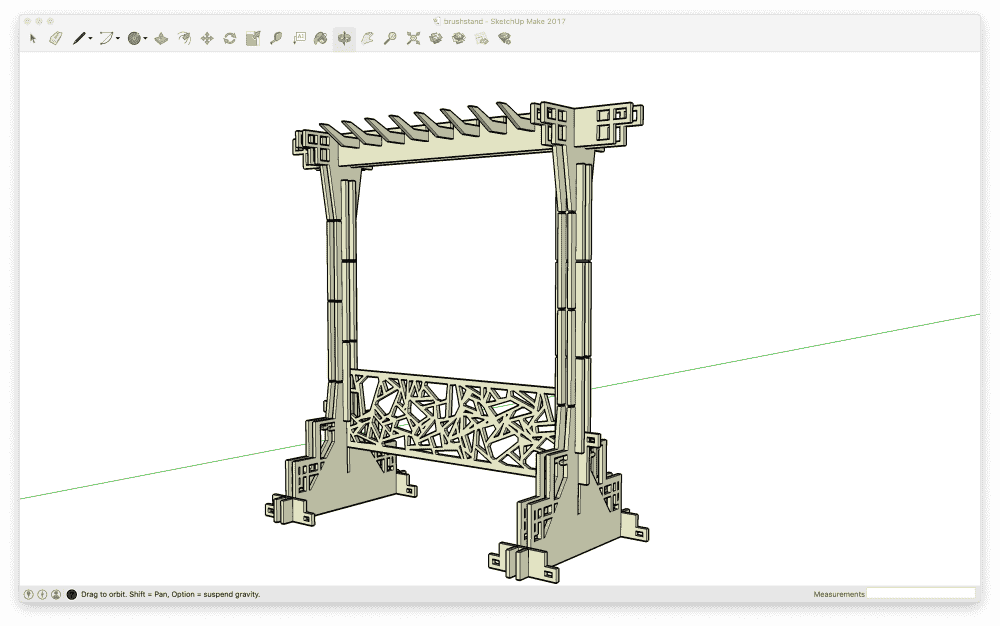I rushed to the CBA lab to print my design. I was very nervous because it was almost the first time I used a laser cutter. (I used one once while I was undergrad, but someone hand-held me and that was a long time ago). However I was strangely confident that I can get it done in 15 minutes. I was very wrong.

The first issue I encountered was that CorelDraw, the software installed in the lab's computer to control the cutter, scaled my SVG weirdly. At first I was unaware, and printed a couple parts that are way too tiny. And my joints didn't fit at all which drove me mad. Then I realized that CorelDraw wasn't treating my SVG's unit as millimetre as I thought it should, but instead as pixel and then computed the scaling with some random ppi/dpi configuration. I couldn't find any SVG import settings in the GUI. I googled and clicked around for a couple minutes, then gave up and used this “dumb trick”: In the SVG I made a 1000x800 box enclosing all the parts, and in CorelDraw I ensure that this box is 1000mm x 800mm by scaling manually. It worked nicely.

Another blunder I committed was that while trying to use the focus tool, I accidentally dropped it into a gap in the machine. After a couple clinking sound the stick was nowhere to be found. I feared that if I were to start cutting then, the stick jammed somewhere would cause the machine to explode and kill everyone. Classmates gathered to help and finally we realized that there was an openable cabinet beneath, where we found the focus tool lying there unscathed. Phew!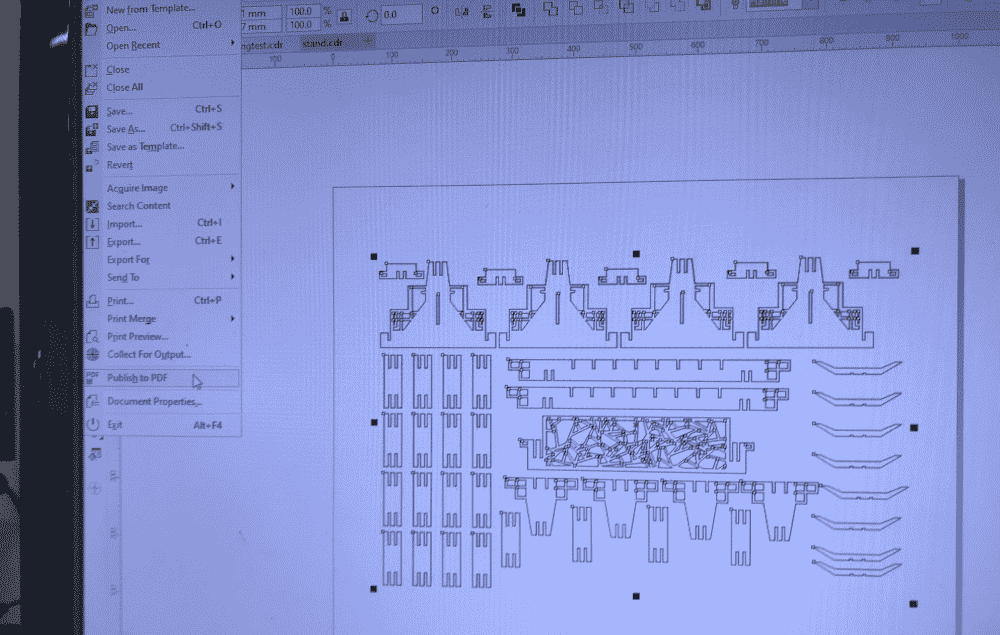I also re-packed the parts in CorelDraw to use space more efficiently, since the cardboard area was smaller than I imagined. After trying a couple different joint clearances as well as laser settings, I found what seemed to be the best combination:

joint clearance 4.1mm
power 100%
speed 2.2%
PPI 215

I printed two joints (from column part of the brush stand). They fitted perfectly, maybe slightly too firmly, but I thought firm is good! Time to print the entire design.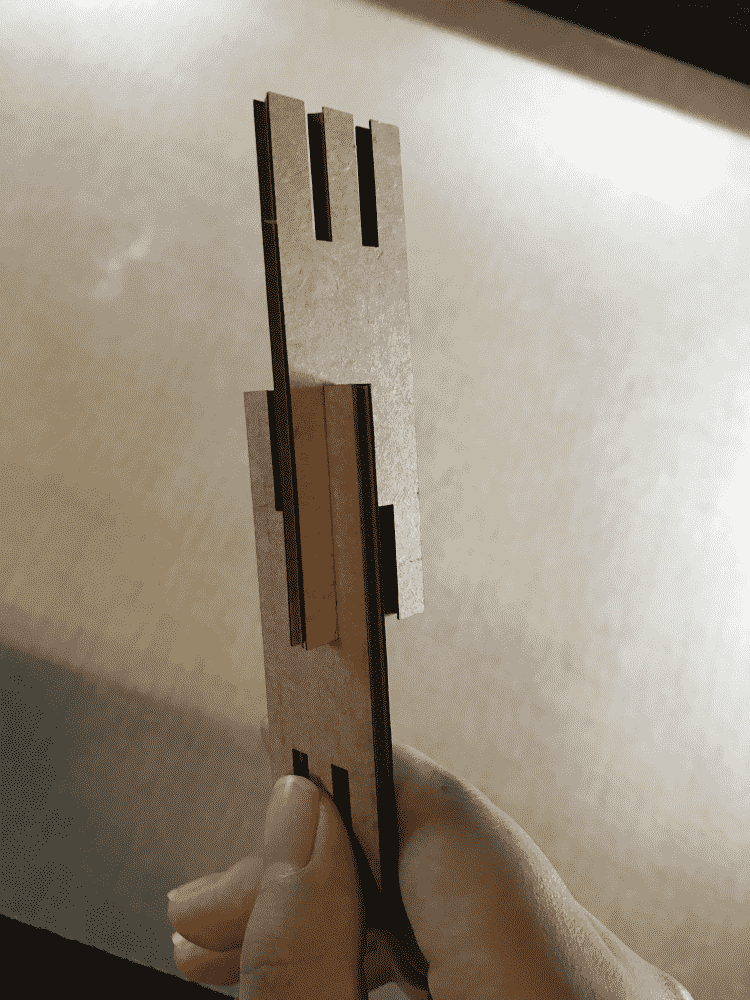It took around half an hour or so for the laser cutter to finish the job. I still overestimated the cardboard area, and one of the parts went off the border and I had to reprint that part.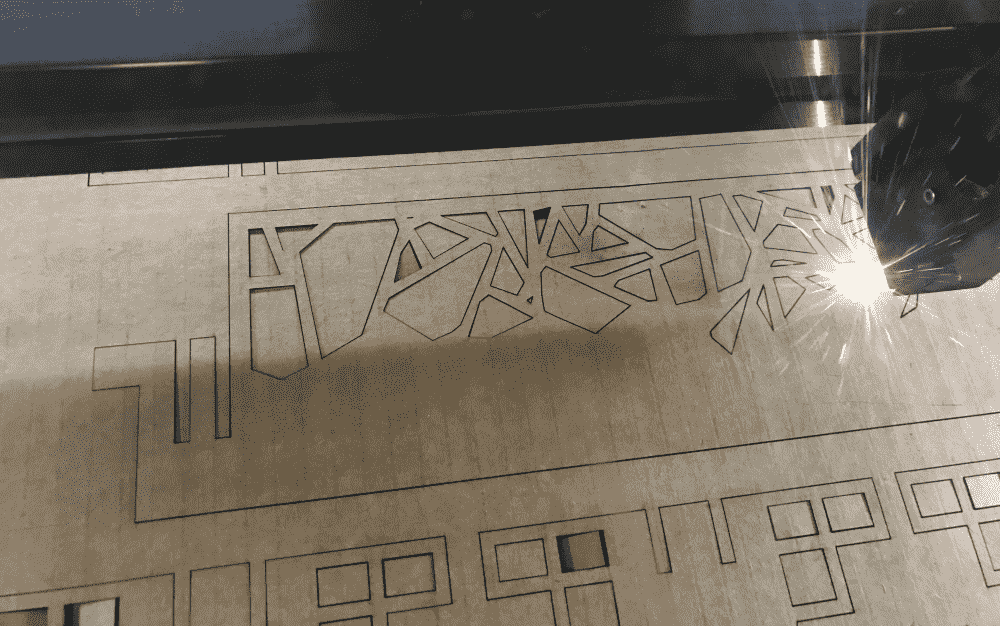The cardboard had uneven thickness and was warped/crooked, which might be the reason why sometimes the laser emitted blazing flames while sometimes it didn't even cut through the board. Nevertheless I thought it went pretty well overall.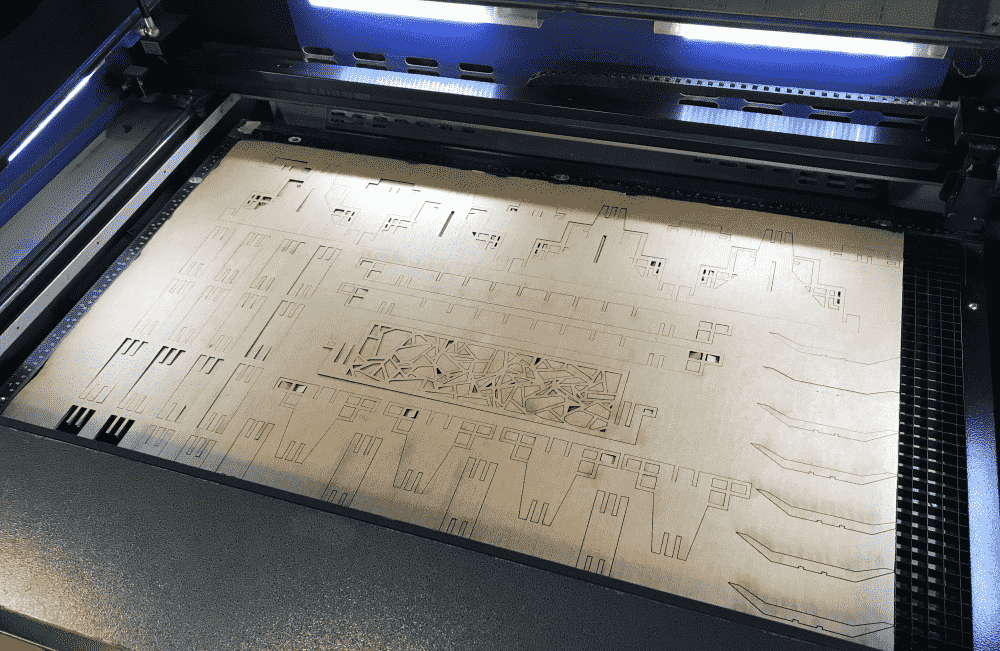I felt very excited to start assembling the pieces. The joints were pretty tight, so sometimes it was a bit difficult to attach them (maybe next time, I should try chamfer joints). But the resultant structure was very robust. I even think that brushes are too light, this stand can probably withstand much heavier stuff.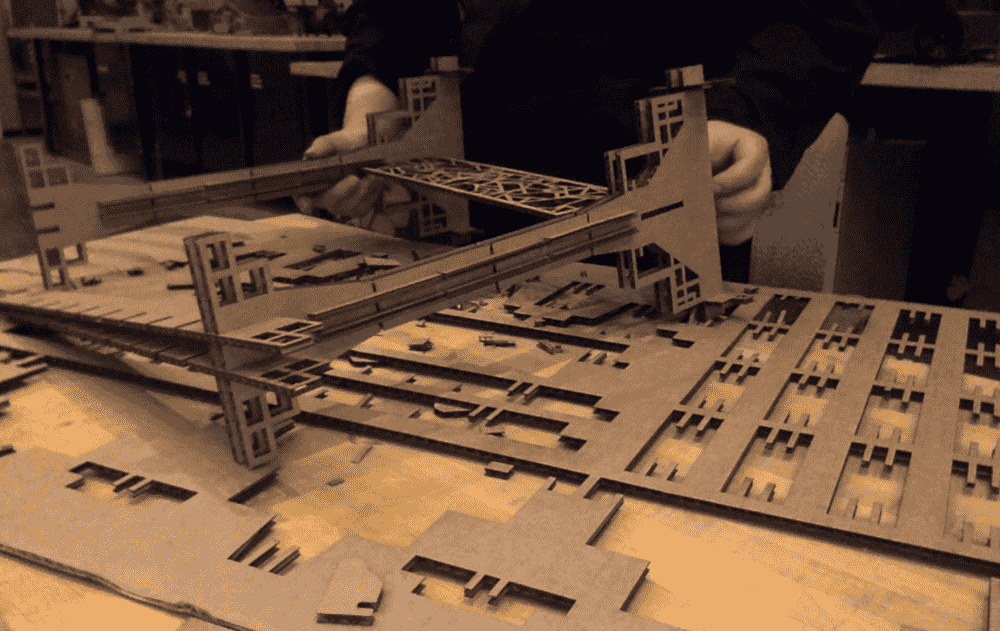A time-lapse of the assembly process:

After assembling the stand I couldn't wait to start hanging my brushes. It worked nicely. Classmates passing by congratulated me, and I was pretty happy too. I think the stand is slightly too large for my tastes (perhaps by 5-10%), but I think the current scale is necessary, since if the skinny parts are skinnier, the cardboard material will start to collapse and structurally fail. Perhaps cardboard is not for tiny delicate things anyway!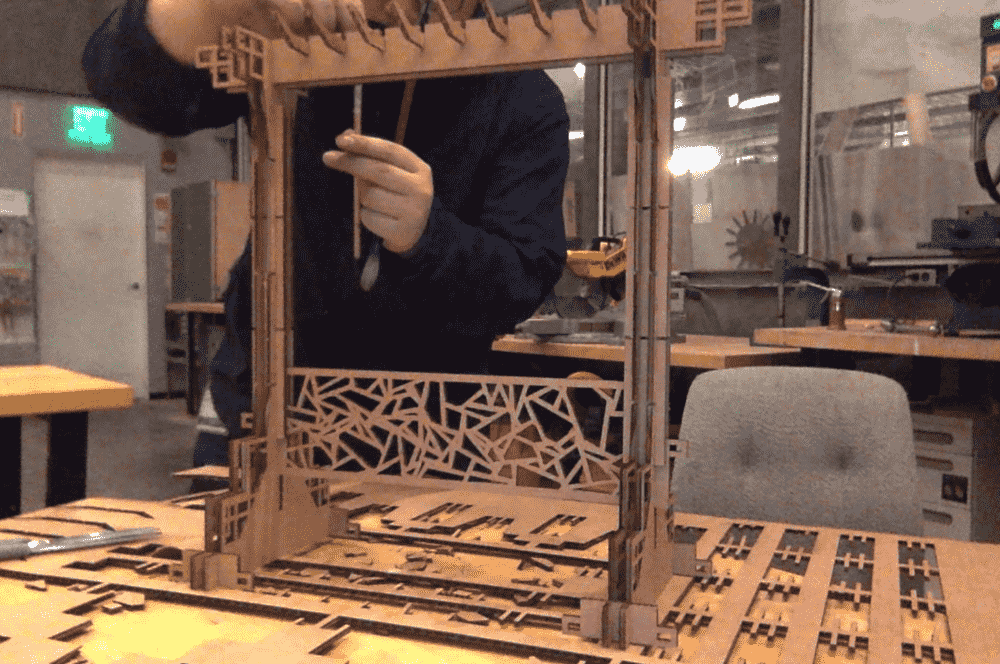A top-down view: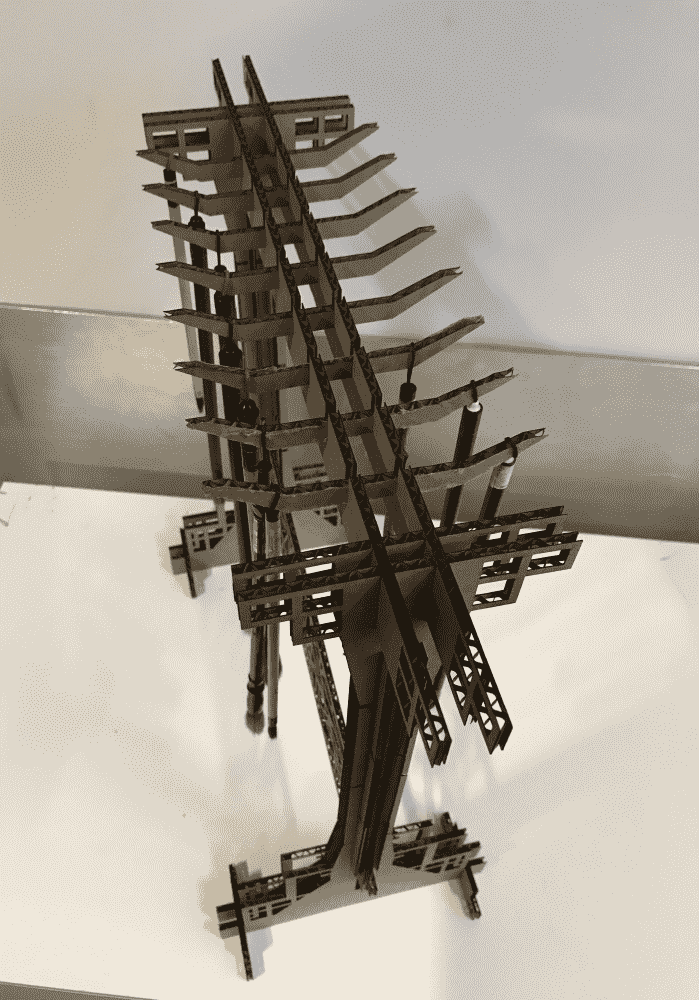I put the brush stand on my studio desk. Nice!